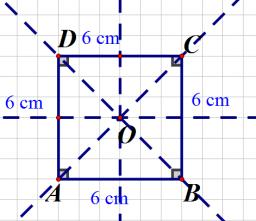# Intersection 3486

The rectangular coordinate system has a point A [-2; -4] and a point S [0; -2]. Determine the coordinates of points B, C, and D so that ABCD is a square and S is the intersection of their diagonals.

b1 =  2
b2 =  -4
c1 =  2
c2 =  0
d1 =  -2
d2 =  0

### Step-by-step explanation:Did you find an error or inaccuracy? Feel free to write us. Thank you!

#### Grade of the word problem:

We encourage you to watch this tutorial video on this math problem: